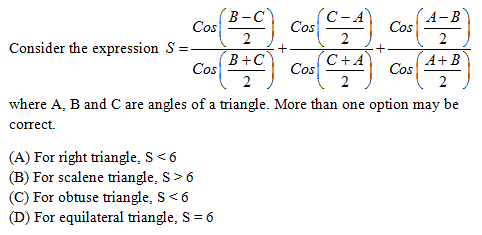# Expression, $S = \sum {{{Cos\left( {{{B - C} \over 2}} \right)} \over {Cos\left( {{{B + C} \over 2}} \right)}}}$# Vector & 3D Classes

Previews of all the 17 classes can be seen below.

# $\int\limits_{ - 1}^2 {\left| {{x^3} - x} \right|} dx$

Evaluate:[CBSE 2012]

Solution

# $\int\limits_0^{ - 2a} {f(x)dx} = .........$

If the system of equations 2x – y + z =0, x- 2y + z = 0 and ax – y + 2z = 0 has infinitely many solutions and f(x) is continuous function satisfying f(x)+f(x+5) = 2, thenis equal to

a) 0
b) 5
c) a
d) –2a

Solution